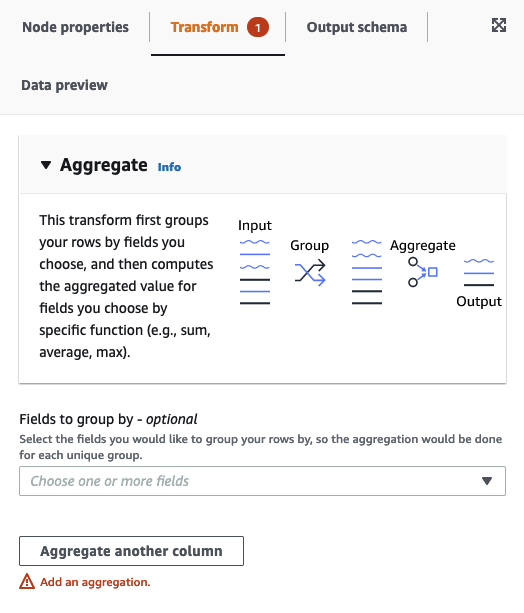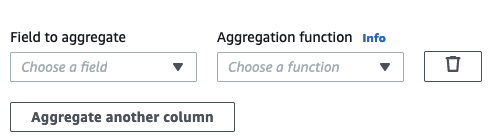Using Aggregate to perform summary calculcations on selected fields - Amazon Glue Studio
Services or capabilities described in Amazon Web Services documentation might vary by Region. To see the differences applicable to the China Regions, see Getting Started with Amazon Web Services in China (PDF).

# Using Aggregate to perform summary calculcations on selected fields

###### To use the Aggregate transform
1. Add the Aggregate node to the job diagram.

2. On the Node properties tab, choose fields to group together by selecting the drop-down field (optional). You can select more than one field at a time or search for a field name by typing in the search bar.

When fields are selected, the name and datatype are shown. To remove a field, choose 'X' on the field.3. Choose Aggregate another column. It is required to select at least one field.4. Choose a field in the Field to aggregate drop-down.

5. Choose the aggregation function to apply to the chosen field:

• avg - calculates the average

• countDistinct - calculates the number of unique non-null values

• count - calculates the number of non-null values

• first - returns the first value that satisfies the 'group by' criteria

• last - returns the last value that satisfies the 'group by' criteria

• kurtosis - calculates the the sharpness of the peak of a frequency-distribution curve

• max - returns the highest value that satisfies the 'group by' criteria

• min - returns the lowest value that satisfies the 'group by' criteria

• skewness - measure of the asymmetry of the probability distribution of a normal distribution

• stddev_pop - calculates the population standard deviation and returns the square root of the population variance

• sum - the sum of all values in the group

• sumDistinct - the sum of distinct values in the group

• var_samp - the sample variance of the group (ignores nulls)

• var_pop - the population variance of the group (ignores nulls)